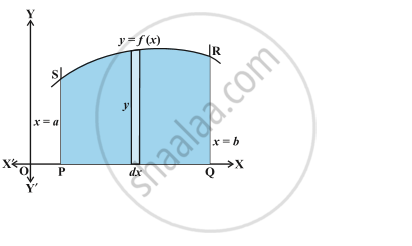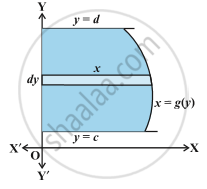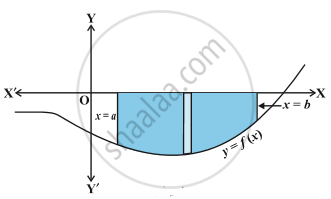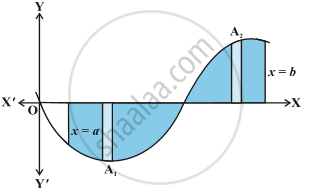# Area Under Simple Curves

#### notes

We consider the easy way of finding  the area bounded by the curve y = f(x), x-axis and the ordinates x = a and x = b. Fig.The area under the curve as composed of large number of very thin vertical strips. Consider an arbitrary strip of height y and width dx, then dA (area of the elementary strip)= ydx, where, y = f(x).
This area is called the elementary area which is located at an arbitrary position within the region which is specified by some value of x between a and b.  The total area A of the region between x-axis, ordinates x = a, x = b and the curve y = f (x) as the result of adding up the elementary areas of thin strips across the region PQRSP. Symbolically, we express
A = int_a^b dA = int_a^b ydx = int_a^b f(x) dx
The area A of the region bounded by the curve x = g (y), y-axis and the lines y  =  c, y = d is given by

A = int_c^d x dy = int_c^d g(y) dy
we consider horizontal strips as shown in the following Fig.Remark:  If the position of the curve under consideration is below the x-axis, then since f (x) < 0 from x = a to x = b, as shown in following  Fig .The area bounded by the curve, x-axis and the ordinates x = a, x = b come out to be negative. But, it is only the numerical value of the area which is taken into consideration. Thus, if the area is negative, we take its absolute value, i.e., |int_a^b f(x) dx|.
Generally, it may happen that some portion of the curve is above x-axis and some is below the x-axis as shown in the following  Fig .Here , A_1 < 0 and A_2> 0.Therefore, the area A bounded by the curve y = f (x), x-axis and the ordinates x = a and x = b is given by A = |A_1|+A_2.

#### description

• Simple curves: lines, parabolas, polynomial functions
If you would like to contribute notes or other learning material, please submit them using the button below.

#### Video Tutorials

We have provided more than 1 series of video tutorials for some topics to help you get a better understanding of the topic.

Series 1

Series 2

Series 3

### Shaalaa.com

Area Bounded by two curves [00:06:41]
S
0%